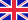•# Statistical theorems

Autor: Source: Wikipedia

Source: Wikipedia. Pages: 50. Chapters: Bayes' theorem, Central limit theorem, Cox's theorem, Shannon-Hartley theorem, Law of large numbers, Gauss-Markov theorem, Ito's lemma, Schuette-Nesbitt formula, Asymptotic equipartition property, Karhunen-Loève theorem,... Viac o knihe

Na objednávku, dodanie 2-4 týždne

15.30 €

bežná cena: 18.00 €

## O knihe

Source: Wikipedia. Pages: 50. Chapters: Bayes' theorem, Central limit theorem, Cox's theorem, Shannon-Hartley theorem, Law of large numbers, Gauss-Markov theorem, Ito's lemma, Schuette-Nesbitt formula, Asymptotic equipartition property, Karhunen-Loève theorem, Berry-Esseen theorem, Rao-Blackwell theorem, Bertrand's ballot theorem, Continuous mapping theorem, Fieller's theorem, Glivenko-Cantelli theorem, Law of total cumulance, Bapat-Beg theorem, Neyman-Pearson lemma, Cochran's theorem, Optional stopping theorem, Schilder's theorem, Wold's theorem, Basu's theorem, Hammersley-Clifford theorem, Law of total variance, Donsker's theorem, Craps principle, Cramér's theorem, Law of the iterated logarithm, Bochner's theorem, Bruck-Chowla-Ryser theorem, Slutsky's theorem, Hájek-Le Cam convolution theorem, Freidlin-Wentzell theorem, Lévy's continuity theorem, Lindeberg's condition, Martingale representation theorem, Law of total covariance, Poisson limit theorem, Stein's lemma, Doob-Meyer decomposition theorem, Laplace principle, Fisher-Tippett-Gnedenko theorem, Varadhan's lemma, Aumann's agreement theorem, Welch-Satterthwaite equation, Frisch-Waugh-Lovell theorem, Le Cam's theorem, Skorokhod's representation theorem, Kolmogorov continuity theorem, Lehmann-Scheffé theorem, Foster's theorem, Raikov's theorem, Robbins lemma. Excerpt: Central limit theorem - a.new,#quickbar a.new/* cache key: enwiki:resourceloader:filter:minify-css:5:f2a9127573a22335c2a9102b208c73e7 */ Central limit theorem This figure demonstrates the central limit theorem. The sample means are generated using a random number generator, which draws numbers between 1 and 100 from a uniform probability distribution. It illustrates that increasing sample sizes result in the 500 measured sample means being more closely distributed about the population mean (50 in this case). It also compares the observed distributions with the distributions that would be expected for a normalized Gaussian distribution, and shows the chi-squared values that quantify the goodness of the fit (the fit is good if the reduced chi-squared value is less than or approximately equal to one). The input into the normalized Gaussian function is the mean of sample means (~50) and the mean sample standard deviation divided by the square root of the sample size (~In probability theory, the central limit theorem (CLT) states conditions under which the mean of a sufficiently large number of independent random variables, each with finite mean and variance, will be approximately normally distributed. The central limit theorem (in its common form) requires the random variables to be identically distributed. Since real-world quantities are often the balanced sum of many unobserved random events, this theorem provides a partial explanation for the prevalence of the normal probability distribution. The CLT also justifies the approximation of large-sample statistics to the normal distribution in controlled experiments. A simple example of the central limit theorem is given by the problem of rolling a large number of identical dice, each of which is weighted unfairly in some unknown way. The distribution of the sum (or average) of the rolled numbers will be well approximated by a

• Vydavateľstvo: Books LLC, Reference Series
• Rok vydania: 2011
• Formát: Paperback
• Rozmer: 246 x 189 mm
• Jazyk: Anglický jazyk
• ISBN: 9781156618004

Generuje redakčný systém BUXUS CMS spoločnosti ui42.